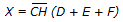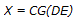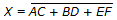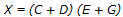# Digital Electronics - Boolean Algebra and Logic Simplification

Exercise : Boolean Algebra and Logic Simplification - General Questions
6.
One of De Morgan's theorems states that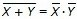. Simply stated, this means that logically there is no difference between:
a NOR and an AND gate with inverted inputs
a NAND and an OR gate with inverted inputs
an AND and a NOR gate with inverted inputs
a NOR and a NAND gate with inverted inputs
Explanation:
No answer description is available. Let's discuss.

7.
The commutative law of Boolean addition states that A + B = A × B.
True
False
Explanation:
No answer description is available. Let's discuss.

8.
Applying DeMorgan's theorem to the expression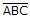, we get ________.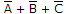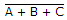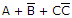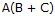Explanation:
No answer description is available. Let's discuss.

9.
The systematic reduction of logic circuits is accomplished by:
using Boolean algebra
symbolic reduction
TTL logic
using a truth table